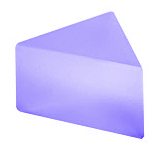Prisms

A prism is a geometric glass figure bounded by planes. These planes are equal polygons oriented in parallel planes. The side faces of a prism are parallelograms. There are triangular, rectangular, hexagonal and some other types of prisms and they are mainly used to deviate beams of light.
To disperse white light into its color components triangular prisms are used. These are triangular prisms with equilateral sides, the angles between adjacent sides of the prism are 60°.prism dispersing white light into the color components triangular prism

The different color components are diffracted in different angles because light changes speed by moving from one medium to another depending on the wavelength (frequency). If light arrives in a straight angle (90 degrees) the light beam moves through the medium in different speed for the different wavelengths but all wavelengths in the same direction. Visible white light is composed of color components from 400 to 700 nm. As soon as the light arrives in an angle different of 90 degrees, the effect of different speed and therefor of different diffraction becomes visible. The components with shorter wavelengths will be further bended then the components with longer wavelengths: red light travels more straight and blue and violet light travels with a stronger change of direction.See: colorslight arriving in an angle of 90°light moves straight through light arriving in an angle smaller 90° components will be diffracted depending on the wavelength

Mostly prisms are used to deflect (re-direct) light by using the internal reflections in the prism. The angles are 45°-90°-45°. If light is arriving at 90° and is only reflected inside the prism without travelling through changing medium the light is not diffracted in components!prisms to re-direct light beams 180° and 90°